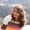Lessons: 20Length: 1.7 hours• Overview
• Transcript

# 4.2 Interoperability

In this lesson, you'll see how you can use Java and Kotlin functions together in the same application. You'll see how to call a Kotlin function from a Java file and vice versa.

## 5.Conclusion1 lesson, 01:17

### 4.2 Interoperability

Hi all. In this lesson we will be finally seeing how we can use Java and Kotlin together in a single project. This is our Kotlin file that we have ben working on from the very beginning. Here is the main function, here I have created a class demo with a function sum in it. Let us remove this class and just have the function. This sum function accepts two parameter, which are of integer type, and returns an integer value. It returns num1 + num2. That is, the sum of the two arguments that it accepts. Let us create a Java file and create a class and a function there. To do that, right click on the Source folder &gt; New &gt; Java Class. JavaDemo, which is our class type, and click on OK. Here we have our JavaDemo.java file created with the class JavaDemo. Let us define the main functions of Java and then let us create another function and call it product. Each one returns the integer type and accept two parameters that will again be integer type. Let us return the product of the incoming arguments. Now, we have a Kotlin file here, and a Java file here. Now let us see how will this FirstKotlinDemo.kt look when the code is compiled. So here we have our Kotlin code in form of class. We already know that this Kotlin file is converted to class and it is named by default as FirstKotlinDemoKt. And then we have the main function here with the area of arguments, of string type. And then, there is the sum function, which will accept two argument and the written type is int, and it returns the sum of two numbers. So, let us first call our Kotlin function from the Java class. How do we do that? This is our Kotlin class, and this is the function inside the FirstKotlinDemoKt class. So in our Java file, we will call the Kotlin function sum. Because it returns a sum we will store the value returned in a variable of integer type. And then we will call the function that is in the FirstKotlinDemoKt.sum and pass two arguments let's say 3 and 7. So here we have int sum, let us print it out here. Is sum, and here we have our sum printed which comes from the Kotlin file. Let us run our Java file, and see what happens. To run the Java file, just right click on the file, and select Run 'Javademo.main'. Because we have the function call in our main function. So here is the output. The Kotlin output is 10. So we have the sum coming from the Kotlin file, which is this function. So we have six is fully called out Kotlin function in our Java file. Now let us call this Java function with this product from our Kotlin file. We have the class JavaDemo, so let us do it. Product which is of integer type, and call our JavaClass, product function and pass the value 3 and 9. Let us run our Kotlin file and see what happens. Select FirstKotlinDemoKt from the drop down and click on Run. So here we are. The Java output is 27, the product of 3 and 9 is 27. So we have successfully called our Java function from our Kotlin file. That's all for this lesson. Happy coding, and keep smiling.

Back to the top